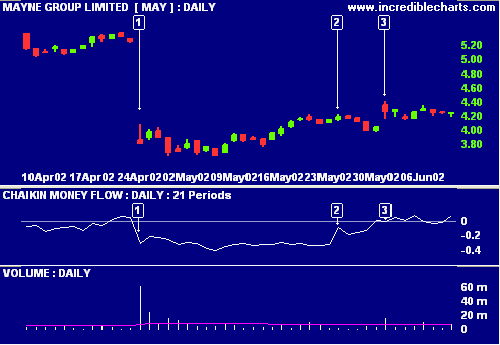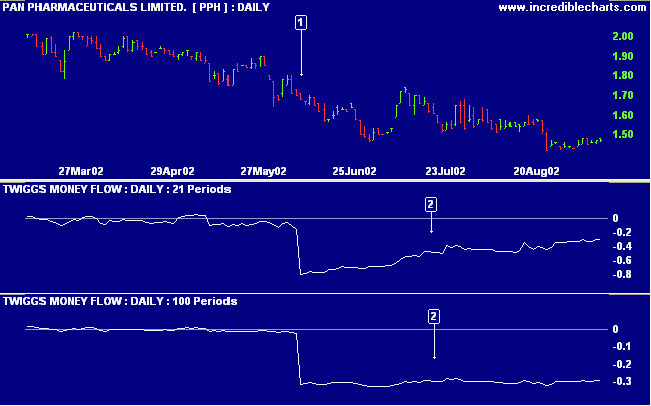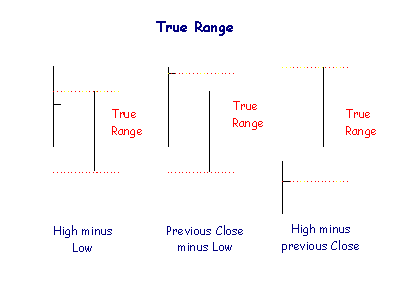# Twiggs® Money Flow

Twiggs Money Flow is Colin Twiggs' derivation of the popular Chaikin Money Flow indicator, which is in turn derived from the Accumulation Distribution line. We are all indebted to Marc Chaikin and Larry Williams for the contribution they have made to the field of technical analysis and price-volume oscillators.

Twiggs Money Flow warns of breakouts and provides useful trend confirmation. It is based on the observation that buying support is normally signaled by:

• increased volume and
• frequent closes in the top half of the daily range.

Likewise, selling pressure is evidenced by:

• increased volume and
• frequent closes in the lower half of the daily range.

After using Chaikin Money Flow intensively for several months, I noticed that the indicator has two weaknesses:

• If price gaps up or down and then closes mid-way between the day's high and low, the indicator records no change. It does not account for gaps.
• CMF also has a tendency to "bark twice". The formula sums Accumulation Distribution for 21 periods and then divides the total by the sum of volume for the identical period. This is very effective in highlighting short- to medium-term accumulation and distribution but leaves the user with the same failing as a simple moving average: unusually high or low data affects the indicator on the day it is added to the indicator period and on the day it is dropped from the calculation. This is far more critical with the money flow indicator than with a moving average and users may witness a surge in the index that is totally unrelated to current price/volume behavior.

### Example

Mayne Nickless (Australia) is plotted with    Chaikin Money Flow and    Volume.Mouse over chart captions to display trading signals.

1. Chaikin Money Flow drops sharply at  as the stock gaps downward on exceptionally high volume, indicating distribution.
2. 22 days later at , Chaikin Money Flow jumps upwards when the data on day  is dropped from the indicator window. There is no unusual volume or price event on day .
3. Price gaps up with strong volume but Chaikin Money Flow dips - because the close is below the mid-point between the day's high and low. The gap up is ignored.

## Two Basic Improvements

Twiggs Money Flow makes two basic improvements to the Chaikin Money Flow formula:

• To solve the problem with gaps, Twiggs Money Flow uses true range, rather than daily Highs minus Lows.
• And, rather than a simple-moving-average-type formula, Twiggs Money Flow applies exponential smoothing, using the method employed by Welles Wilder for many of his indicators.

### Example

Mayne Nickless (Australia) is plotted with    Twiggs Money Flow and    Chaikin Money Flow.Mouse over chart captions to display trading signals.

1. The stock gaps downward at  on exceptionally high volume. Twiggs Money Flow and Chaikin Money Flow drop sharply, indicating distribution.
2. There is no unusual volume or event 22 days later at , reflected by the smooth indicator line on Twiggs Money Flow. By contrast, Chaikin Money Flow jumps up sharply at  when day  data is dropped from the indicator calculation.
3. Price gaps up on day  with above-average volume. Twiggs Money Flow rises because of the upward gap while Chaikin MF dips (because the Close is below the mid-point between the day's High and Low).

## Twiggs® Money Flow Trading Signals

Twiggs Money Flow signals accumulation if above zero, while negative values signal distribution. The higher the reading (above or below zero), the stronger the signal.

• Go long if a breakout above resistance is supported by Twiggs Money Flow above zero.
• Go short if a breakout below support is confirmed by negative Twiggs Money Flow.

Divergences also provide good signals:

• Go long on a bullish divergence.
• Go short on a bearish divergence.

The strongest confirmation of the above signals is when either:

• Twiggs Money Flow is trending upwards and completes a trough without crossing below zero, or
• Twiggs Money Flow trends downwards and completes a peak without crossing above zero.

In other words: when Twiggs Money Flow respects the zero line.

### Example

Flight Centre (Australia) with    150-day and    21-day Exponential Moving Averages and    Twiggs Money Flow.Mouse over chart captions to display trading signals.

1. Go long at  on bullish divergence.
2. Twiggs Money Flow has respected the zero line, forming a trough above zero; strong confirmation of the earlier bullish divergence.
3. Go long at  on breakout above the resistance level (new 6-month high). Twiggs Money Flow has barely crossed below zero in the 10 months prior to the breakout - an exceptionally strong accumulation signal.

### Volume Spikes

Beware of large volume spikes - on Twiggs MF or Chaikin MF. A gentle slope back towards zero is not a divergence but is caused by the exponential smoothing: the indicator will tend towards zero, over time, in the absence of other factors. Where there is any doubt about a divergence, check with a longer-term Twiggs MF indicator.

### Example

Pan Pharmaceuticals with    21-day Twiggs Money Flow and    100-day Twiggs Money Flow.Mouse over chart captions to display trading signals.

1. A large volume spike (more than 40 times average daily volume), while PPH is trending lower, causes Twiggs Money Flow to drop sharply.
2. The gradual upward slope on the 21-day Twiggs MF over the next three months is not a bullish divergence but the result of exponential smoothing: the indicator tends towards zero, over time, in the absence of other factors. This is best illustrated by the longer-term 100-day Twiggs Money Flow which remains almost completely flat over the same period.

### Setup

See Indicator Panel for directions on how to set up Twiggs Money Flow. The default setting is:

• 21 days

To alter the default settings - Edit Indicator Settings.

### True Range

True Range is the greater of:

• High for the period less the Low for the period.
• High for the period less the Close for the previous period.
• Close for the previous period and the Low for the current period.Basically, True Range High (TRH) is the greater of today's High and yesterday's Close.

True Range Low (TRL) is the lesser of today's Low and yesterday's Close.

## Twiggs® Money Flow Formula

Twiggs Money Flow substitutes true range for the daily high and low and then applies exponential smoothing, separately, to the sum of Accumulation Distribution and the divisor:

1. #### Calculate True Range High and True Range Low:

True Range High (TRH) is the greater of:

• High [today] and
• Closing price [yesterday]

True Range Low (TRL) is the lesser of:

• Low [today] and
• Closing price [yesterday]
2. #### Calculate Accumulation Distribution (AD) using True Range High and True Range Low:

AD = { (Close - TRL) - (TRH - Close) } / {TRH - TRL} * Volume

3. #### Apply exponential smoothing* to AD:

• Calculate AD as the sum of AD for the first 21 days
• Repeat this process for each subsequent day**.
* Welles Wilder's Indicators

TMF uses Welles Wilder's formula for calculating an exponential moving average. Wilder not use the standard exponential moving average formula. See Wilder Moving Average.
A 14-day Wilder moving average is equivalent to a 27-day exponential moving average using the standard formula.

1. #### Do the same with the divisor:

• Calculate V as the sum of volume for the same 21 day period as in 3. above
• On the next day, multiply V by 20/21 and add Volume for day 22
• Repeat this process for each subsequent day**
** Exponential Smoothing

Some observant readers have questioned why Step 3 is not divided by 21 days, to create an exponential moving average. The same applies to Step 4.
You will note that Step 5 divides the result of Step 3 by Step 4. Division of the numerator (Step 3) and the denominator (Step 4) by 21 is therefore redundant: the one offsets the other.

This leads to another question: Why are AD (Step 3) and Volume (Step 4) not divided by 21?
If we take Step 4 as an example, V is the sum of 21 days of volume. If we want to add the next days Volume, we must remove one days Volume from V, to keep the total constant at 21 days. We do this by multiplying V by the fraction 20/21.

1. #### Divide AD by V:

Twiggs Money Flow = AD / V expressed as a percentage.

## MetaStock® Formula

Twiggs Money Flow
https://www.incrediblecharts.com/indicators/twiggs_money_flow.php

{2009-10-15}
periods:=Input("TMF periods",1,100,21);
TRH:=Max(Ref(C,-1),H);
TRL:=Min(Ref(C,-1),L);
TR:=TRH-TRL;